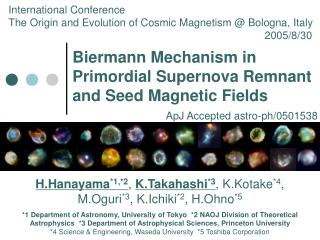DownloadDownload PresentationBiermann Mechanism in Primordial Supernova Remnant and Seed Magnetic Fields

# Biermann Mechanism in Primordial Supernova Remnant and Seed Magnetic Fields

Download Presentation## Biermann Mechanism in Primordial Supernova Remnant and Seed Magnetic Fields

- - - - - - - - - - - - - - - - - - - - - - - - - - - E N D - - - - - - - - - - - - - - - - - - - - - - - - - - -
##### Presentation Transcript

1. International Conference The Origin and Evolution of Cosmic Magnetism @ Bologna, Italy 2005/8/30 Biermann Mechanism in Primordial Supernova Remnant and Seed Magnetic Fields ApJ Accepted astro-ph/0501538 H.Hanayama*1,*2, K.Takahashi*3, K.Kotake*4, M.Oguri*3, K.Ichiki*2, H.Ohno*5 *1 Department of Astronomy, University of Tokyo *2 NAOJ Division of Theoretical Astrophysics *3 Department of Astrophysical Sciences, Princeton University *4 Science & Engineering, Waseda University *5 Toshiba Corporation

2. Origin of cosmic magnetic fields • Magnetic fields (~μG) are ubiquitous in the universe, but the origin is not yet clear. • Various mechanisms have been suggested as a possible source of the seed fields. • Cosmological origin : • electro-weak or QCD phase transition, Inflation, CMB second-order perturbation • Astrophysical origin : • first stars, protogalaxy, AGN, reionization • Basically, the astrophysical magnetogenesis invokes the Biermann mechanism (Biermann 1950).

3. Electric current Magnetic field n1 > n2 |Vx2| > |Vx1| Biermann Mechanism • The Biermann Mechanism is an induction process of magnetic fields by the vorticity of the electric currents. • The vorticity is produced by the spatial gradient of the electron pressure not parallel to that of the density. Development of vorticity y -∇p/n1 ∇ρ -∇p/n2 x ∇p

4. Biermann term • The amplitude of the magnetic field produced by the Biermann mechanism can be estimated as • This generates magnetic fields from zero in the region which non-adiabatic process, such as shock wave, works. • In this research, we studied magnetgenesis of shock wave in Primordial Supernova Remnant (SNR) as the astrophysical origin. , .

5. Our Works • 2D-MHD simulation of primordial supernova remnant • Induction equation with Biermann term • Calculation is started from initial condition B0=0. • Inhomogeneous ISM • Inhomogeneity with scale length λ, average density n0, and explosion energy E0 are varied. • Analytic estimation; • the generation process of magnetic field in shock front is estimated analytically, and compared to calculation results. • Further, we evaluated the seed fields from the total magnetic energy and the primordial star formation rate.

6. Model & Calculation ~2D cylindrical coordinates~ • Inhomogeneous ISM (Bromm et al. 2003) • n = n0×2±1 cm-3 (n0=0.2-10cm-3), T0 = 104 K • Inhomogeneity with the scale length λ (λ=1-10 pc) • Pair-instability supernova of 250 M8 (Fryer et al. 2001) • Ionized gas with radiative cooling 1200 grid × 1200 grid 120 pc × 120 pc Inhomogeneous ISM Z (pc) Z Calculate region Density R Supernova explosion E0 = 1052 or 1053 erg R (pc)

7. MHD equation Mass conservation Momentum conservation Energy conservation Induction equation Biermann term Λ0(T) & Γ0 : radiative cooling function (collisional ionization equilibrium) and heating coefficient χ = 5/6 : ionization fraction , Scheme : Modified Lax-Wendroff Code : CANS (Coordinated Astronomical Numerical Software) produced by Ryoji Matsumoto (Chiba Univ.) & Takaaki Yokoyama (Tokyo Univ.)

8. Results of Numerical Simulations Center; 10-14 G Average; 10-15 G • 1.3×105 yrs after the explosion • At the end of Sedov phase n | | Density Magnetic Field

9. Time evolution of the total magnetic energy explosion energy E0 erg , average ISM density n0 cm-3, scale length λ pc In every model, the total magnetic energy is 1028-31 erg.

10. Order estimation of magnetic field We applied ram pressure as the pressure of the shock front. , We obtain L : a scale length of the pressure component perpendicular to the density gradient .

11. Total magnetic energy The magnetic energy per each primordial supernova remnant can be estimated as . This is consistent with the value obtained from our numerical simulations.

12. Star formation rate (SFR) • Pello et al., 2004 • Search of distant • Galaxy using • cluster lensing • Candidate of about • ten Galaxies @ z • > 7 is discovered. • The star formation • rate is estimated • in consideration of • various uncertainty. Log( SFR ) Red shift (z)

13. Is it candidate for the seed fields? Magnetic energy density produced by primordial supernova remnant :fγγ is mass fraction of first stars (Schneider et al. 2002) Sufficient for the seed fields Magnetic energy density required for the seed fields (Athreya et al. 1998) the seed fields of galactic dynamo

14. Conclusion • We studied the generation of magnetic fields by Biermann Mechanism in primordial supernova remnant. • The amplitude of generated magnetic fields is 10-15 [G] @z~10. • The total magnetic energy is 1030 [erg] @ 100 [pc]. • The magnetic energy density in consideration of the star formation rate is evaluated as eB ~ 10-40 [erg cm-3]. • eB is larger than the required energy density 10-42 [erg cm-3] @B = 10-20 [G], and enough for the seed fields. • Primordial supernova remnant is a promising source for the seed fields!

15. n [cm-3] T [K] p [cm-3K] |Bφ| [G]